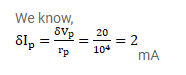# The dynamic plate resistance of a triode value is

Question:

The dynamic plate resistance of a triode value is $10 \mathrm{k}^{\Omega}$. Find the change in the plate current if the plate voltage is changed from $200 \mathrm{~V}$ to $220 \mathrm{~V}$.

Solution: